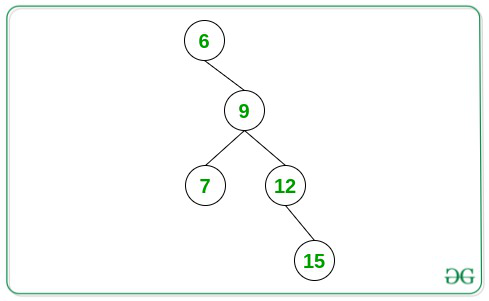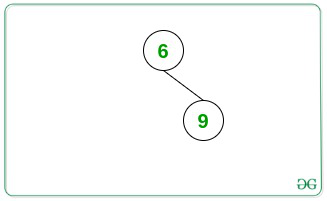# Longest path to the bottom of a Binary Tree forming an Arithmetic Progression

• Difficulty Level : Medium
• Last Updated : 20 Aug, 2021

Given a Binary Tree consisting of N nodes, the task is to find the length of the longest path from any node to the bottom of the tree such that all the node values form an Arithmetic Progression.

Examples:

Input:Output: 4
Explanation:
From the above given tree, the longest path with node values forming an AP is {6, 9, 12, 15} (Length = 4).

Input:Output: 2

Approach: The given problem can be solved by using recursion and performing the DFS Traversal on the given tree. The idea is to keep track of the difference between the current root node and the next descendant node and update the length of the longest path accordingly. Follow the steps below to solve the given problem:

• Initialize a variable, say maxLength as 1 that stores the maximum length of the path from any node to the bottom of the tree forming an Arithmetic Progression.
• Define a function, say dfs(root, currentDifference, count, maxLength) that takes the current root node, current difference, count of nodes forming AP, and the resultant maximum length as the parameter and perform the following steps:
• If the root’s left node exists, then perform the following steps:
• Find the difference between the value of the root and its left node.
• If the difference is found to be currentDifference, then update the value of maxLength to the maximum of maxLength and (count + 1) and recursively call for the function dfs(root->left, currentDifference, count + 1, maxLength).
• Otherwise, recursively call for the dfs(root->left, difference, 2, maxLength).
• If the root’s right node exists, then perform the following steps:
• Find the difference between the value of the root and its right node.
• If the difference is found to be currentDifference, then update the value of maxLength to the maximum of maxLength and (count + 1) and recursively call for the function dfs(root->right, currentDifference, count + 1, maxLength).
• Otherwise, recursively call for the dfs(root->left, difference, 2, maxLength).
• If the left child of the given root node exists, then call for the dfs(root->left, difference, 2, maxLength) where the difference is the difference between the value of the root and its left node.
• If the right child of the given root node exists, then call for the dfs(root->right, difference, 2, maxLength) where the difference is the difference between the value of the root and its right node.
• After completing the above steps, print the value of maxLength as the resultant maximum length of the path from any node to the bottom of the tree forming an Arithmetic Progression.

Below is the implementation of the above approach:

## C++

 `// C++ program for the above approach` `#include "bits/stdc++.h"``using` `namespace` `std;` `// Structure of the Tree Node``struct` `Tree {``    ``int` `val;``    ``Tree *left, *right;``};` `// Function to create a new node``Tree* newNode(``int` `data)``{``    ``Tree* temp = ``new` `Tree();``    ``temp->val = data;``    ``temp->left = temp->right = NULL;``    ``return` `temp;``}` `// Function to perform DFS Traversal``// to find the maximum length of a path``// to a bottom node forming an AP``void` `dfs(Tree* root, ``int` `currentDifference,``         ``int` `count, ``int``& maxLength)``{``    ``// If the root's left child exists``    ``if` `(root->left) {` `        ``// Calculate the difference``        ``int` `difference = root->left->val``                         ``- root->val;` `        ``// If the difference is same``        ``// as the current difference``        ``if` `(difference == currentDifference) {``            ``dfs(root->left, currentDifference,``                ``count + 1, maxLength);` `            ``// Update the maxLength``            ``maxLength = max(maxLength,``                            ``count + 1);``        ``}` `        ``// Otherwise``        ``else` `{``            ``dfs(root->left, difference,``                ``2, maxLength);``        ``}``    ``}` `    ``// If the root's right child exists``    ``if` `(root->right) {` `        ``// Find the difference``        ``int` `difference = root->right->val``                         ``- root->val;` `        ``// If the difference is the same``        ``// as the current difference``        ``if` `(difference == currentDifference) {` `            ``dfs(root->right, currentDifference,``                ``count + 1, maxLength);` `            ``// Update the maxLength``            ``maxLength = max(maxLength,``                            ``count + 1);``        ``}` `        ``// Otherwise``        ``else` `{``            ``dfs(root->right, difference,``                ``2, maxLength);``        ``}``    ``}``}` `// Function to find the maximum length``// of the path from any node to bottom``// of the tree forming an AP``int` `maximumLengthAP(Tree* root)``{` `    ``// Base Cases``    ``if` `(root == NULL)``        ``return` `0;` `    ``if` `(root->left == NULL``        ``and root->right == NULL) {``        ``return` `1;``    ``}` `    ``// Stores the resultant``    ``// maximum length of the path``    ``int` `maxLength = 2;` `    ``// If the root's left child exists``    ``if` `(root->left) {` `        ``int` `difference = root->left->val``                         ``- root->val;``        ``dfs(root->left, difference, 2,``            ``maxLength);``    ``}` `    ``// If the root's right child exists``    ``if` `(root->right) {``        ``int` `difference = root->right->val``                         ``- root->val;``        ``dfs(root->right, difference, 2,``            ``maxLength);``    ``}` `    ``// Return the maximum length obtained``    ``return` `maxLength;``}` `// Driver Code``int` `main()``{` `    ``// Given Tree``    ``Tree* root = newNode(6);``    ``root->right = newNode(9);``    ``root->right->left = newNode(7);``    ``root->right->right = newNode(12);``    ``root->right->right->right = newNode(15);` `    ``cout << maximumLengthAP(root);` `    ``return` `0;``}`

## Java

 `// Java program for the above approach``import` `java.lang.*;``import` `java.util.*;` `class` `GFG{``    ` `static` `int` `maxLength;   ``    ` `// TreeNode class``static` `class` `Node``{``    ``public` `int` `val;``    ``public` `Node left, right;``};` `static` `Node newNode(``int` `key)``{``    ``Node temp = ``new` `Node();``    ``temp.val = key;``    ``temp.left = temp.right = ``null``;``    ``return` `temp;``}` `// Function to perform DFS Traversal``// to find the maximum length of a path``// to a bottom node forming an AP``static` `void` `dfs(Node root, ``int` `currentDifference,``                ``int` `count)``{``    ` `    ``// If the root's left child exists``    ``if` `(root.left != ``null``)``    ``{``        ` `        ``// Calculate the difference``        ``int` `difference = root.left.val - root.val;` `        ``// If the difference is same``        ``// as the current difference``        ``if` `(difference == currentDifference)``        ``{``            ``dfs(root.left, currentDifference,``                ``count + ``1``);` `            ``// Update the maxLength``            ``maxLength = Math.max(maxLength,``                                 ``count + ``1``);``        ``}` `        ``// Otherwise``        ``else``        ``{``            ``dfs(root.left, difference, ``2``);``        ``}``    ``}` `    ``// If the root's right child exists``    ``if` `(root.right != ``null``)``    ``{``        ` `        ``// Find the difference``        ``int` `difference = root.right.val - root.val;` `        ``// If the difference is the same``        ``// as the current difference``        ``if` `(difference == currentDifference)``        ``{``            ``dfs(root.right, currentDifference,``                ``count + ``1``);` `            ``// Update the maxLength``            ``maxLength = Math.max(maxLength,``                                 ``count + ``1``);``        ``}` `        ``// Otherwise``        ``else``        ``{``            ``dfs(root.right, difference, ``2``);``        ``}``    ``}``}` `// Function to find the maximum length``// of the path from any node to bottom``// of the tree forming an AP``static` `int` `maximumLengthAP(Node root)``{``    ` `    ``// Base Cases``    ``if` `(root == ``null``)``        ``return` `0``;` `    ``if` `(root.left == ``null` `&&``        ``root.right == ``null``)``    ``{``        ``return` `1``;``    ``}` `    ``// Stores the resultant``    ``// maximum length of the path``     ``maxLength = ``2``;` `    ``// If the root's left child exists``    ``if` `(root.left != ``null``)``    ``{``        ``int` `difference = root.left.val - root.val;``        ``dfs(root.left, difference, ``2``);``    ``}` `    ``// If the root's right child exists``    ``if` `(root.right != ``null``)``    ``{``        ``int` `difference = root.right.val - root.val;``        ``dfs(root.right, difference, ``2``);``    ``}` `    ``// Return the maximum length obtained``    ``return` `maxLength;``}   ` `// Driver code``public` `static` `void` `main(String[] args)``{` `    ``// Given Tree``    ``Node root = newNode(``6``);``    ``root.right = newNode(``9``);``    ``root.right.left = newNode(``7``);``    ``root.right.right = newNode(``12``);``    ``root.right.right.right = newNode(``15``);``    ` `    ``System.out.println(maximumLengthAP(root));``}``}` `// This code is contributed by offbeat`

## Python3

 `# Python3 program for the above approach``maxLength ``=` `2` `class` `Node:``  ` `    ``# Constructor to set the data of``    ``# the newly created tree node``    ``def` `__init__(``self``, key):``        ``self``.val ``=` `key``        ``self``.left ``=` `None``        ``self``.right ``=` `None` `# Function to perform DFS Traversal``# to find the maximum length of a path``# to a bottom node forming an AP``def` `dfs(root, currentDifference, count):``    ` `    ``global` `maxLength` `    ``# If the root's left child exists``    ``if` `(root.left !``=` `None``):``        ``# Calculate the difference``        ``difference ``=` `root.left.val ``-` `root.val` `        ``# If the difference is same``        ``# as the current difference``        ``if` `(difference ``=``=` `currentDifference):``        ` `            ``dfs(root.left, currentDifference, count ``+` `1``)` `            ``# Update the maxLength``            ``maxLength ``=` `max``(maxLength, count ``+` `1``)``        `  `        ``# Otherwise``        ``else``:``            ``dfs(root.left, difference, ``2``)` `    ``# If the root's right child exists``    ``if` `(root.right !``=` `None``):``        ``# Find the difference``        ``difference ``=` `root.right.val ``-` `root.val` `        ``# If the difference is the same``        ``# as the current difference``        ``if` `(difference ``=``=` `currentDifference):``        ` `            ``dfs(root.right, currentDifference, count ``+` `1``)` `            ``# Update the maxLength``            ``maxLength ``=` `max``(maxLength, count ``+` `1``)` `        ``# Otherwise``        ``else``:``            ``dfs(root.right, difference, ``2``)` `# Function to find the maximum length``# of the path from any node to bottom``# of the tree forming an AP``def` `maximumLengthAP(root):` `    ``global` `maxLength``    ` `    ``# Base Cases``    ``if` `(root ``=``=` `None``):``        ``return` `0` `    ``if` `(root.left ``=``=` `None` `and` `root.right ``=``=` `None``):``        ``return` `1` `    ``# If the root's left child exists``    ``if` `(root.left !``=` `None``):``        ``difference ``=` `root.left.val ``-` `root.val``        ``dfs(root.left, difference, ``2``)` `    ``# If the root's right child exists``    ``if` `(root.right !``=` `None``):``        ``difference ``=` `root.right.val ``-` `root.val``        ``dfs(root.right, difference, ``2``)` `    ``# Return the maximum length obtained``    ``return` `maxLength` `# Given Tree``root ``=` `Node(``6``)``root.right ``=` `Node(``9``)``root.right.left ``=` `Node(``7``)``root.right.right ``=` `Node(``12``)``root.right.right.right ``=` `Node(``15``)``  ` `print``(maximumLengthAP(root))` `# This code is contributed by decode2207.`

## C#

 `// C# program for the above approach``using` `System;` `class` `GFG{``    ` `static` `int` `maxLength;   ``    ` `// TreeNode class``class` `Node``{``    ``public` `int` `val;``    ``public` `Node left, right;``};` `static` `Node newNode(``int` `key)``{``    ``Node temp = ``new` `Node();``    ``temp.val = key;``    ``temp.left = temp.right = ``null``;``    ``return` `temp;``}` `// Function to perform DFS Traversal``// to find the maximum length of a path``// to a bottom node forming an AP``static` `void` `dfs(Node root, ``int` `currentDifference,``                ``int` `count)``{``    ` `    ``// If the root's left child exists``    ``if` `(root.left != ``null``)``    ``{``        ` `        ``// Calculate the difference``        ``int` `difference = root.left.val - root.val;` `        ``// If the difference is same``        ``// as the current difference``        ``if` `(difference == currentDifference)``        ``{``            ``dfs(root.left, currentDifference,``                ``count + 1);` `            ``// Update the maxLength``            ``maxLength = Math.Max(maxLength,``                                 ``count + 1);``        ``}` `        ``// Otherwise``        ``else``        ``{``            ``dfs(root.left, difference, 2);``        ``}``    ``}` `    ``// If the root's right child exists``    ``if` `(root.right != ``null``)``    ``{``        ` `        ``// Find the difference``        ``int` `difference = root.right.val - root.val;` `        ``// If the difference is the same``        ``// as the current difference``        ``if` `(difference == currentDifference)``        ``{``            ``dfs(root.right, currentDifference,``                ``count + 1);` `            ``// Update the maxLength``            ``maxLength = Math.Max(maxLength,``                                 ``count + 1);``        ``}` `        ``// Otherwise``        ``else``        ``{``            ``dfs(root.right, difference, 2);``        ``}``    ``}``}` `// Function to find the maximum length``// of the path from any node to bottom``// of the tree forming an AP``static` `int` `maximumLengthAP(Node root)``{``    ` `    ``// Base Cases``    ``if` `(root == ``null``)``        ``return` `0;` `    ``if` `(root.left == ``null` `&&``        ``root.right == ``null``)``    ``{``        ``return` `1;``    ``}` `    ``// Stores the resultant``    ``// maximum length of the path``     ``maxLength = 2;` `    ``// If the root's left child exists``    ``if` `(root.left != ``null``)``    ``{``        ``int` `difference = root.left.val - root.val;``        ``dfs(root.left, difference, 2);``    ``}` `    ``// If the root's right child exists``    ``if` `(root.right != ``null``)``    ``{``        ``int` `difference = root.right.val - root.val;``        ``dfs(root.right, difference, 2);``    ``}` `    ``// Return the maximum length obtained``    ``return` `maxLength;``}   ` `// Driver code``public` `static` `void` `Main(String[] args)``{``    ` `    ``// Given Tree``    ``Node root = newNode(6);``    ``root.right = newNode(9);``    ``root.right.left = newNode(7);``    ``root.right.right = newNode(12);``    ``root.right.right.right = newNode(15);``    ` `    ``Console.WriteLine(maximumLengthAP(root));``}``}` `// This code is contributed by gauravrajput1`

## Javascript

 ``

Output:

`4`

Time Complexity: O(N)
Auxiliary Space: O(1)

My Personal Notes arrow_drop_up